# Tutorial 19: Universal Introduction

Logical System

2013

### Skill to be acquired:

To learn the rule for adding a Universal Quantifier.

Bergmann The Logic Book Section 10.1.

### The Tutorial

There also is a rule for adding a Universal Quantifier (often called 'Universal Generalization'). This permits the step illustrated by the following proof fragments.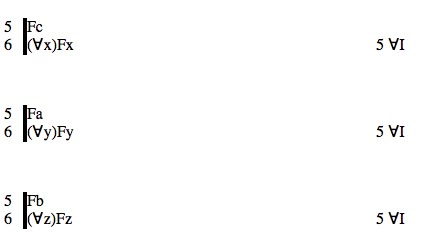The rule is

#### The Rule of Universal Introduction UI

If a derivation contains a line of the form

n. <<scope>[<constant>/<variable>]> <any justification>

then a line of the form

(∀<variable>)<scope> 'n ∀I'

may be added to the derivation, provided that

a) the <constant> does not occur in the <assumptions> of the formula that is generalized (the formula of line n), and
b) the <constant> does not occur in (∀<variable>)<scope>.

This is very similar to doing the reverse of Universal Elimination, or doing Universal Elimination backwards. The first line, the one generalized on, is an instantiation of the second (for example, in the first of the above illlustrations, the second line is (∀x)Fx and the first line is Fc --ie in thought you could get the first by Universal Elimination from the second).

You will notice that there are restrictions on the rule.

Let us see if we can motivate these. At first glance, inferences like those illustrated above seem just plain wrong: it does not follow from the fact that a is F that everything is F. But, actually, the above inferences do not show that. Here is a derivation that shows it, or would show it if it were correctThis is an attempt at the inference Fa ∴ (∀x)Fx (ie the inference 'a is F therefore everything is F). But, if you remember the vertical line notation used in our derivations (explained in Sentential Tutorial 6), Fa is an assumption of line 1 (and line 2, for that matter), so the constant 'a' appears in an assumption of line where Universal Introduction is being attempted. Restriction (a) -- the <constant> does not occur in the <assumptions> of the formula that is generalized (the formula of line n) -- rules this out.

Well, you may wonder, how can we get lines the have formulas like Fa but yet those lines do not have 'a' among the assumptions?

Here is a way: from a prior elimination of a Universal Quantifier.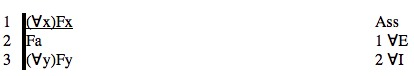This is a perfectly good derivation of (∀x)Fx ∴ (∀y)Fy.

The second restriction also prevents invalid inferences. The remark was made above that a Universal Introduction step is like Universal Elimination in reverse. It is, but not entirely. Look at these three Universal Eliminations and attempted Universal Introductions.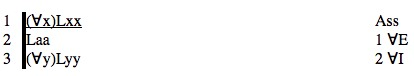This is good. We haven't met two place predicates or relations before, but a relation like Lxy might symbolize the English 'x loves y'. Then what the premise of the argument says is 'Everybody loves himself (or herself)' and the conclusion says the same thing but using a different variable of quantification. But what about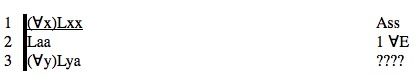Here, in thought line 2 could have been obtained in reverse from line 3 by Universal Elimination (ie it is a substitution instance). But the premise says 'Everybody loves himself (or herself)' and the conclusion says 'Everybody loves a' where 'a' is a constant ie it stands for an object, perhaps Arthur, so the conclusion really might be 'Everybody loves Arthur'. But that does not follow from the premise! What stops this is restriction (b): the <constant> does not occur in (∀<variable>)<scope>. The constant 'a' does occur in line 3, so this step is not permitted.

Similarly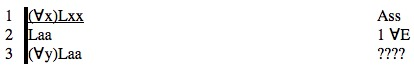Line 3 is not permitted because the constant 'a' occurs in the universally quantified formula.

There will be more on these restrictions in Tutorial 23.

## Exercise to accompany Predicate Tutorial 9

### Exercise 1(of 1)

Derive the following

(a) Fa ∴ (∀x)Fa /*This cannot be proved, however the theorem prover does it. That is a mistake, the theorem prover either has a sense of humour or has not studied the rules carefully enough.*/
(b) (∀x) (Fx&Gx) ∴ (∀x)Fx
(c) (∀x) (Fx&Gx) ∴ (∀y)Fy
(d) ∴ (∀x)Fx≡(∀y)Fy
(e) (∀x) (Fx&Gx) ∴ (∀y)Fy&(∀z)Gz
(f) ∴ (∀x) (Fx&Gx) ≡ (∀y)Fy&(∀z)Gz
(g) ∴ (∀x) (Fx&Gx) ≡ (∀x)Fx&(∀x)Gx

If you decide to use the web application for the exercises you can launch it from here Deriver[Bergmann] — username 'logic' password 'logic'. Then either copy and paste the above formulas into the Journal or use the Deriver File Menu to Open Web Page with this address https://softoption.us/test/easyDeriver/CombinedExercisesEasyDBergmann.html .

Preferences

You may need to set some Preferences for this.

• you can check that the parser is set to bergmann.